# What’s new? November 2019

2019-20: Sep | Oct#### Enrichment sessions 9 and 10

We have added resources for Enrichment sessions 9 and 10.

Session 9, titled Proof, looks at giving students a flavour of some interesting proofs in a manner accessible to relatively young students. Having encountered the idea of rational and irrational numbers in Enrichment session 8, students should be able to appreciate the proof that there exist to irrational numbers, $$x$$ and $$y$$, such that $$x^{y}$$ is irrational—and see how this proof is similar to a seemingly unrelated logic puzzle. This session also covers the famous problem of the bridges of Königsberg and includes an interactive applet.

Session 10 gives students a whistle-stop tour of various ideas relating to elections and voting systems, including pluralities, majorities, vote splitting, cardinal voting, ranked voting, Arrow’s Impossibility Theorem, transitivity, constituencies and how UK general elections and US presidential elections are run, as well as the concept of gerrymandering (drag the slider below):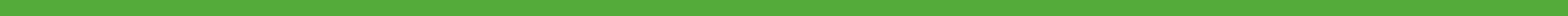#### Update to A17b – Solving linear equations in one unknown algebraically where the unknown is on both sides of the equation

We have added a second set of slides for this topic dealing with equations with algebraic fractions. Below are the Alpha, Beta, and Gamma exercises from this new set of slides.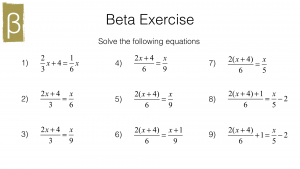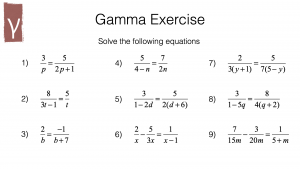We have also added an applet to show the effects of applying operations to both sides of an equation: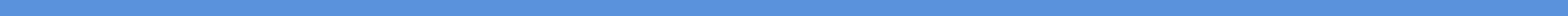#### TopTopics and Random Question Generators

Questions on sequences have been added to TopTopics: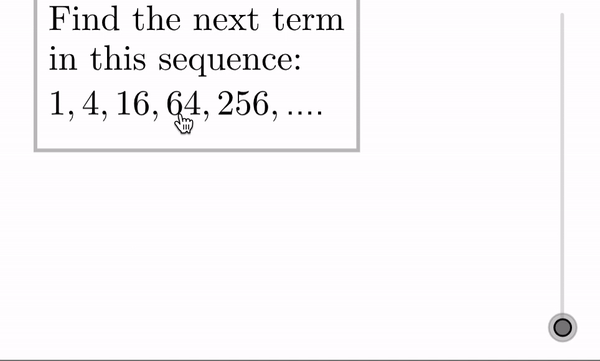We have included quick-access sets of random question generators under the Teacher Resources for the following sequences-related topics: A23a, A24b, A24c, A25a (three levels of difficulty), and A25b (three levels of difficulty).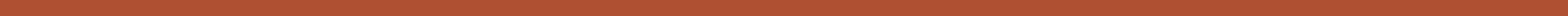#### Names of polygons

We have added this applet to topic G1a that shows students the general names of polygons with up to 13 sides:

Drag the slider across to see the names of polygons with up to 13 sides.

See G4a – Properties of special triangles and quadrilaterals for more on particular types of triangles and quadrilaterals.